Function Repository Resource:

# IsogonalConjugate

Get the isogonal conjugate of a point with respect to a triangle or tetrahedron

Contributed by: Ed Pegg Jr
 ResourceFunction["IsogonalConjugate"][simplex,p] gives the isogonal conjugate of point p with respect to the triangle or tetrahedron simplex.

## Details

The simplex may be a triangle defined by three 2D points, a tetrahedron defined by four 3D points or an n-simplex defined by n+1 points.
Given a triangle ΔABC, the isogonal conjugate of P is constructed by reflecting the lines PA, PB and PC about the angle bisectors and taking the intersection.
Given a tetrahedron ABCD, the isogonal conjugate of P is constructed by reflecting the lines PA, PB, PC and PD about the angle bisectors of the dihedral edges and taking the intersection.
In triangle ΔABC, the trilinear coordinates of a point are the ratio a:b:c of signed distances to the sides. The trilinear coordinates of the isogonal conjugate are the ratio a-1:b-1:c-1.
In tetrahedron ABCD, the barycentric coordinates of a point are the ratio of signed volumes of the four tetrahedra points by replacing a vertex with the point a:b:c:d. Let ΔA be the squared face area of ΔBCD. The barycentric coordinates of the isogonal conjugate are then ΔA/a:ΔB/b:ΔC/c:ΔD/d.

## Examples

### Basic Examples (2)

Compute the isogonal conjugate of a point with respect to a given triangle:

 In:=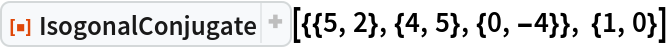Out=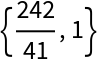Find the isogonal conjugate of a point with respect to a triangle:

 In:=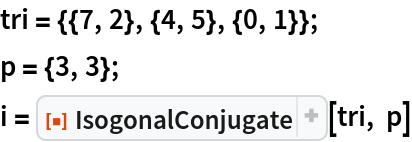Out=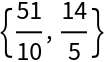The isogonal conjugate of the isogonal conjugate is the original point:

 In:=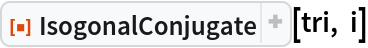Out=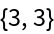Show the triangle, point (green) and isogonal conjugate (blue):

 In:=Out=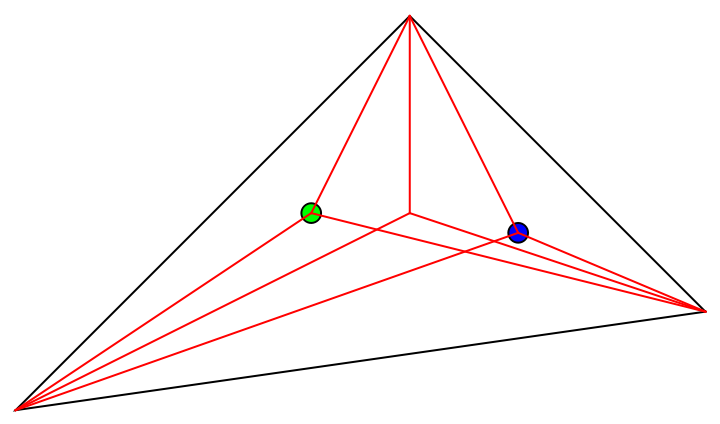The centers of the incircle and excircles are the only four self-conjugate points:

 In:=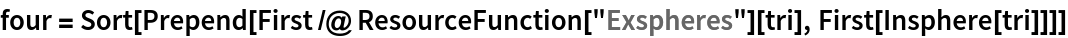Out=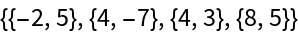In:=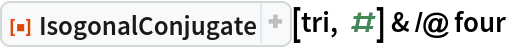Out=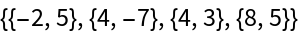In:=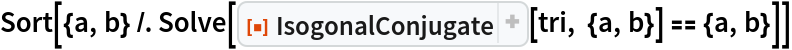Out=The product of trilinear coordinates for a point and isogonal conjugate gives three identical values:

 In:=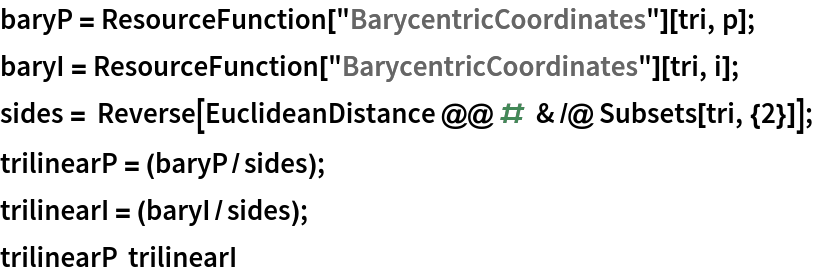Out=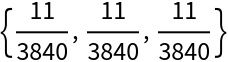### Scope (3)

Compute the isogonal conjugate of a Point with respect to a Triangle:

 In:=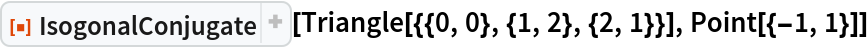Out=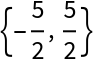Compute the isogonal conjugate of a Point with respect to a Tetrahedron:

 In:=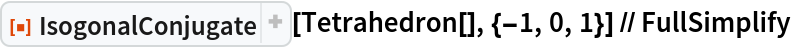Out=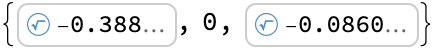Compute the isogonal conjugate of a 5D point with respect to a simplex:

 In:=Out=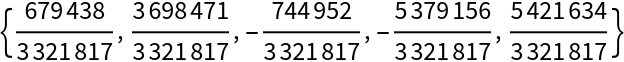Find the Monge point of a semi-random tetrahedron:

 In:=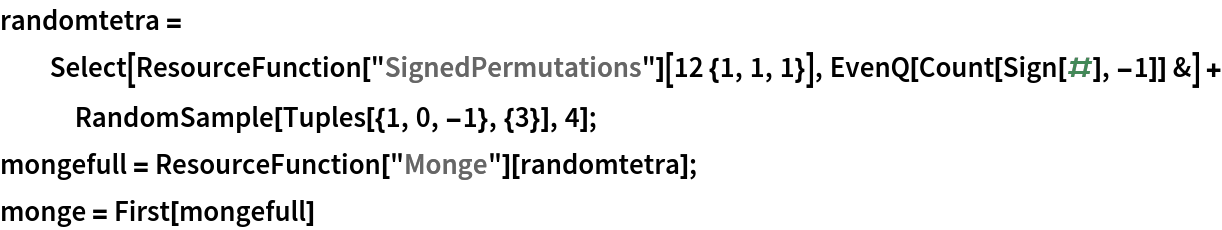Out=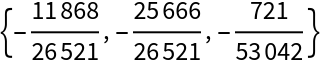Find the isogonal conjugate of the Monge point:

 In:=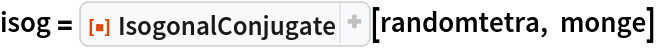Out=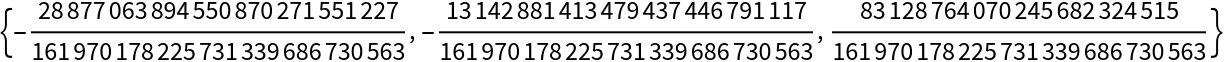The circumsphere of the face reflections of a point is centered at the isogonal conjugate (and vice-versa):

 In:=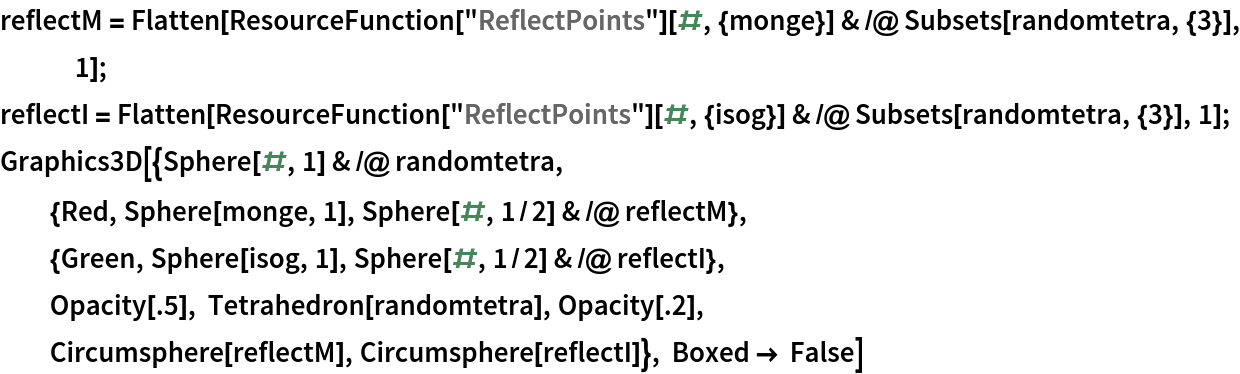Out=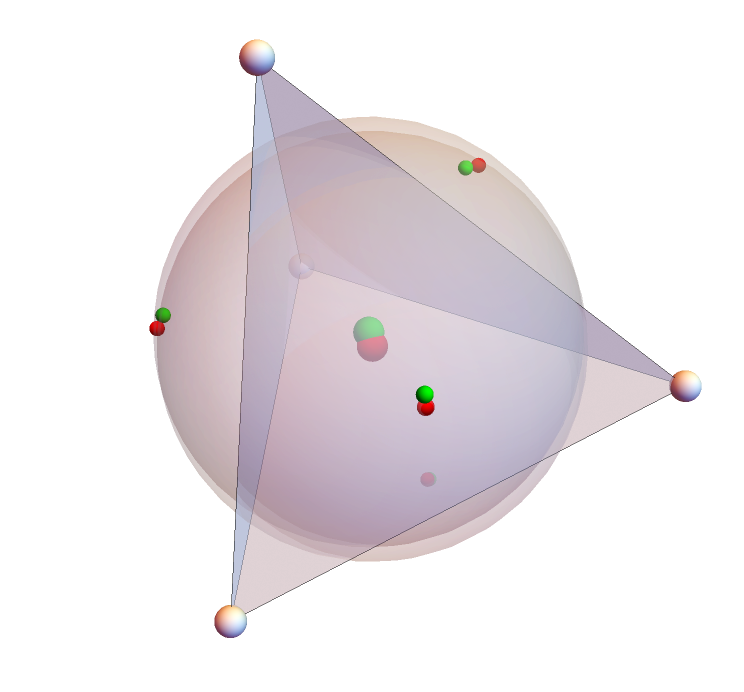### Neat Examples (2)

For a tetrahedron, calculate the five insphere and exsphere points:

 In:=Out=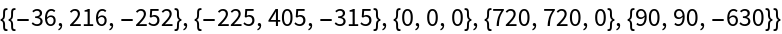These five points are all equal to their isogonal conjugates:

 In:=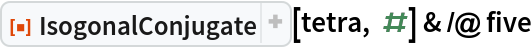Out=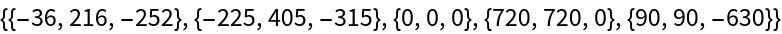Three other points are their own isogonal conjugate in a tetrahedron:

 In:=Out=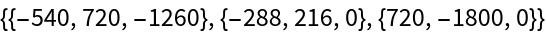Show the 16 collinear sets of three points formed by four tetrahedral points and eight self-conjugates:

 In:=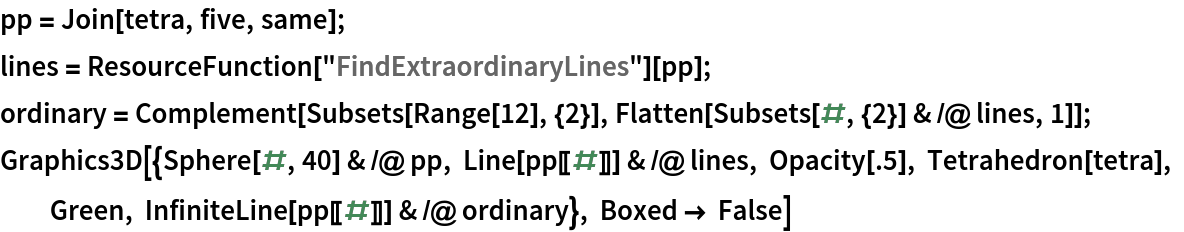Out=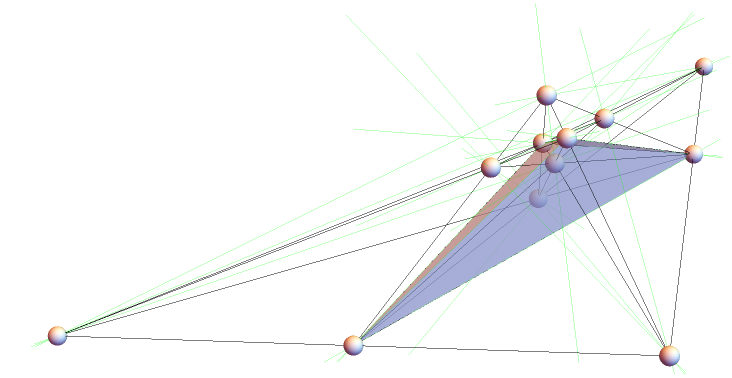Find intersection points and repeat to obtain 50 lines:

 In:=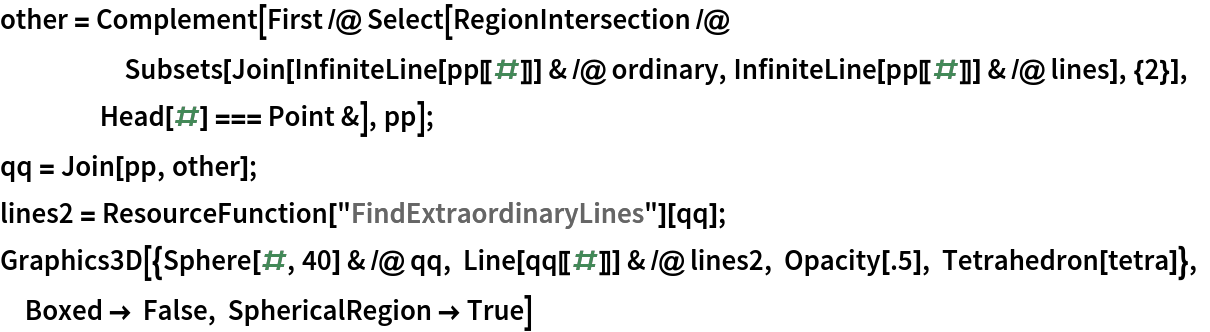Out=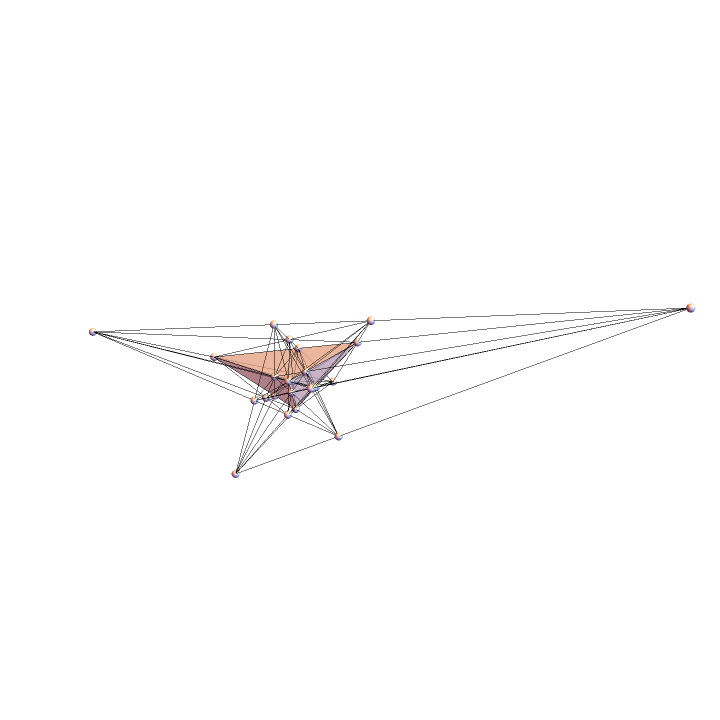The following triangle has nice Euler line points for the circumcenter, centroid, nine-point center and orthocenter:

 In:=Out=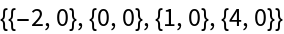The Jerabek hyperbola is the locus of the isogonal conjugates of points on the Euler line. Use GroebnerBasis to derive the implicit equation:

 In:=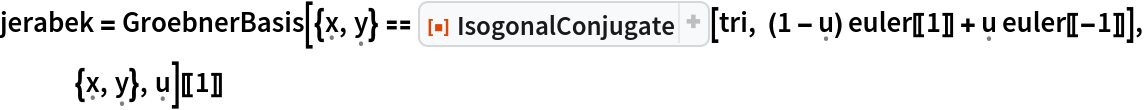Out=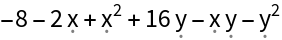The isogonal conjugates of the Euler points are the orthocenter, symmedian, Kosnita point and circumcenter:

 In:=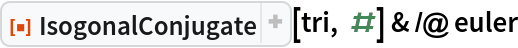Out=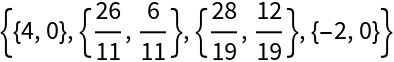A graphic of the triangle with the Euler line (blue), circumcenter|circumcircle|perpendicular bisectors (red), centroid|medians (cyan), nine-point center|circle (brown) and orthocenter|altitudes (green) and the isogonal conjugate of the Euler line, the Jerabek hyperbola:

 In:=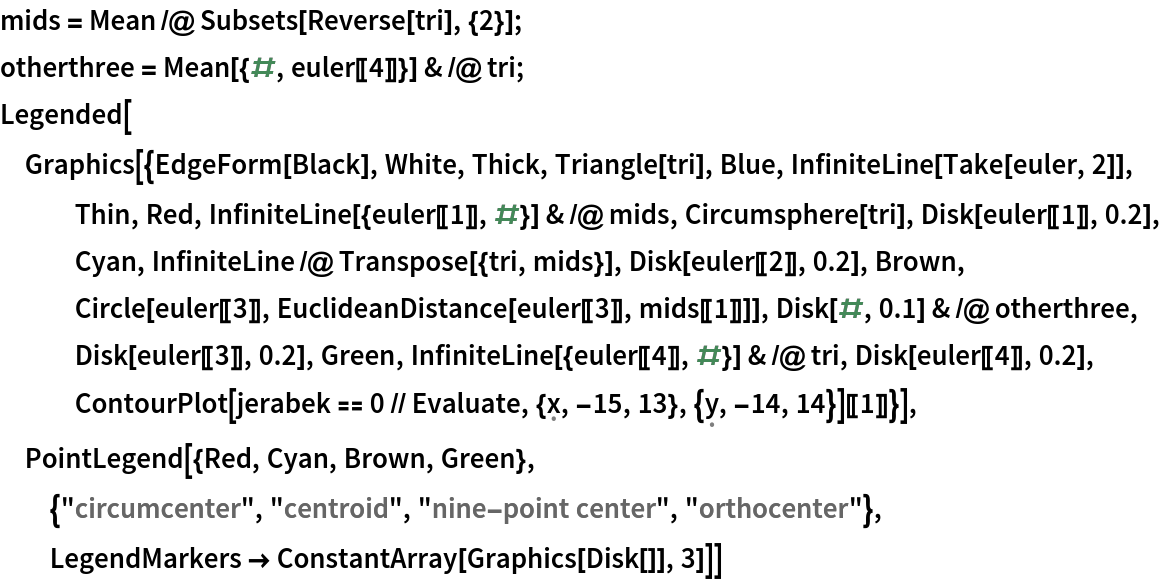Out=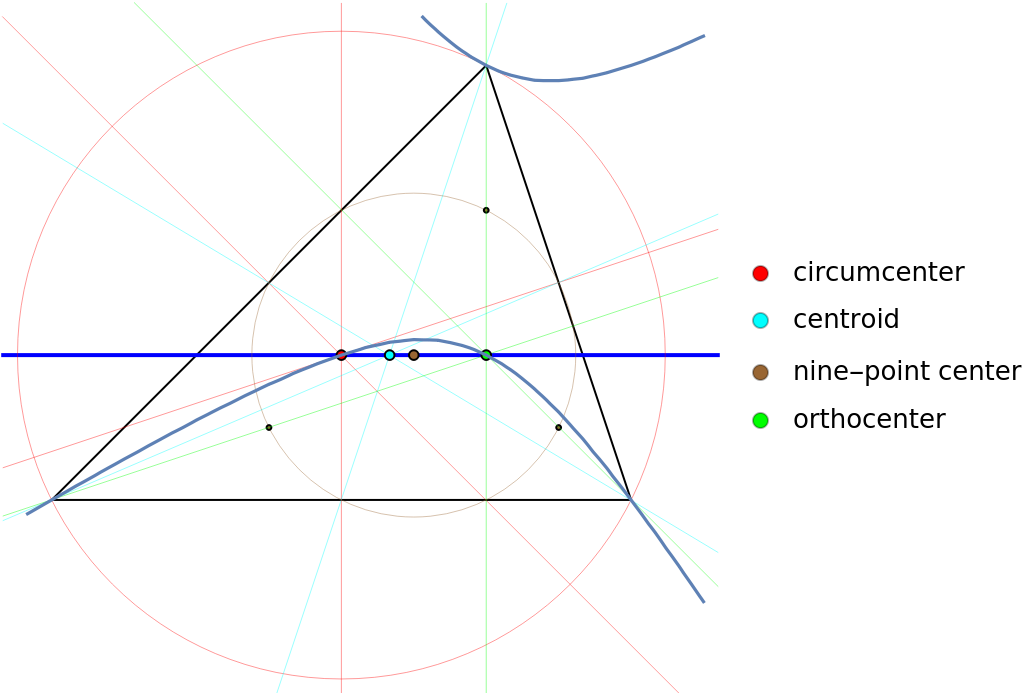## Version History

• 1.1.0 – 22 August 2022
• 1.0.0 – 19 August 2022